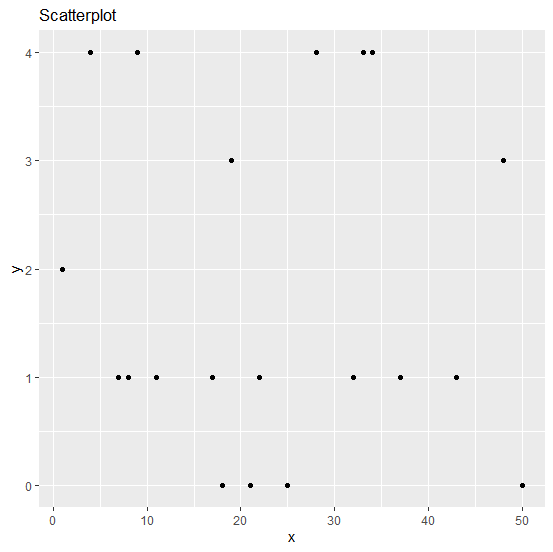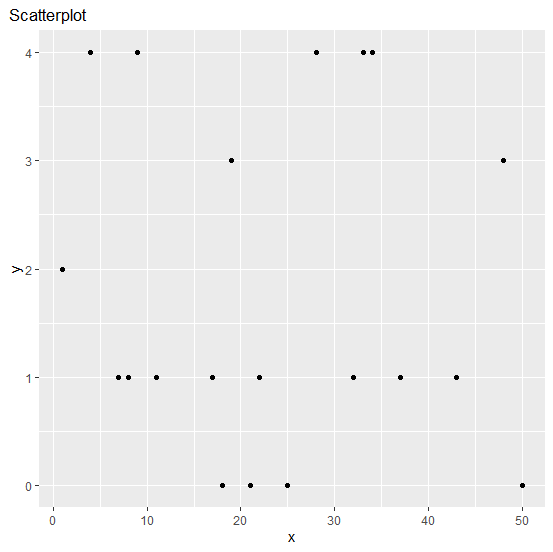# How to change the adjustment of the plot title using ggplot2 to align it above the Y-axis labels in R?

R ProgrammingServer Side ProgrammingProgramming

When we create a plot and put a title above it, the alignment of the title is left-adjusted by default and that lies on the edge of the plot area. But sometimes, we want to display the title on the above side of the Y-axis labels, therefore, we can use theme function and set the hjust argument accordingly.

## Example

Consider the below data frame −

Live Demo

x<-sample(1:50,20)
y<-rpois(20,2)
df<-data.frame(x,y)
df

## Output

   x  y
1  1  2
2  50 0
3  19 3
4  33 4
5  43 1
6  17 1
7  7  1
8  28 4
9  18 0
10 22 1
11 21 0
12 11 1
13 34 4
14  9 4
15  4 4
16 37 1
17 48 3
18 25 0
19  8 1
20 32 1

## Example

library(ggplot2)
ggplot(df,aes(x,y))+geom_point()+labs(title="Scatterplot")

## Output## Example

ggplot(df,aes(x,y))+geom_point()+labs(title="Scatterplot")+theme(plot.title=element_tex
t(hjust=-0.07))

## Output